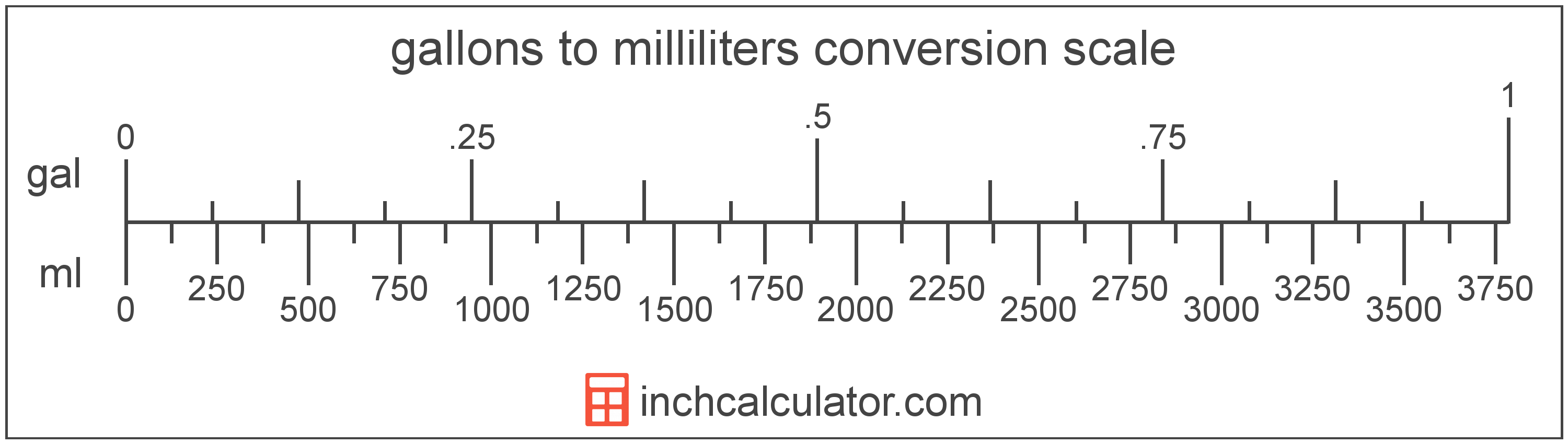# Milliliters to Gallons Converter

Enter the volume in milliliters below to get the value converted to gallons.

Results in Gallons:1 mL = 0.000264 gal

Do you want to convert gallons to milliliters?

## How to Convert Milliliters to Gallons

To convert a measurement in milliliters to a measurement in gallons, multiply the volume by the following conversion ratio: 0.000264 gallons/milliliter.

Since one milliliter is equal to 0.000264 gallons, you can use this simple formula to convert:

gallons = milliliters × 0.000264

The volume in gallons is equal to the volume in milliliters multiplied by 0.000264.

For example, here's how to convert 5,000 milliliters to gallons using the formula above.
gallons = (5,000 mL × 0.000264) = 1.32086 gal## What Is a Milliliter?

The milliliter is a unit of volume equal to 1 cubic centimeter, 1/1,000 of a liter, or about 0.061 cubic inches.

The milliliter is an SI unit of volume in the metric system. In the metric system, "milli" is the prefix for thousandths, or 10-3. A milliliter is sometimes also referred to as a millilitre. Milliliters can be abbreviated as mL, and are also sometimes abbreviated as ml or mℓ. For example, 1 milliliter can be written as 1 mL, 1 ml, or 1 mℓ.

Since one milliliter is equivalent to one cubic centimeter, milliliters are sometimes expressed using the abbreviation for a cubic centimeter (cc) for things such as medical dosages or engine displacements.

## What Is a Gallon?

The US liquid gallon is a unit of fluid volume equal to four quarts, eight pints, or sixteen cups. The US liquid gallon should not be confused with the US dry gallon or the imperial gallon, which are different units of measure.

The gallon is a US customary unit of volume. Gallons can be abbreviated as gal; for example, 1 gallon can be written as 1 gal.

## Milliliter to Gallon Conversion Table

Table showing various milliliter measurements converted to gallons.
Milliliters Gallons
1 mL 0.000264 gal
2 mL 0.000528 gal
3 mL 0.000793 gal
4 mL 0.001057 gal
5 mL 0.001321 gal
6 mL 0.001585 gal
7 mL 0.001849 gal
8 mL 0.002113 gal
9 mL 0.002378 gal
10 mL 0.002642 gal
20 mL 0.005283 gal
30 mL 0.007925 gal
40 mL 0.010567 gal
50 mL 0.013209 gal
60 mL 0.01585 gal
70 mL 0.018492 gal
80 mL 0.021134 gal
90 mL 0.023775 gal
100 mL 0.026417 gal
200 mL 0.052834 gal
300 mL 0.079252 gal
400 mL 0.105669 gal
500 mL 0.132086 gal
600 mL 0.158503 gal
700 mL 0.18492 gal
800 mL 0.211338 gal
900 mL 0.237755 gal
1,000 mL 0.264172 gal
2,000 mL 0.528344 gal
3,000 mL 0.792516 gal
4,000 mL 1.0567 gal
5,000 mL 1.3209 gal
6,000 mL 1.585 gal
7,000 mL 1.8492 gal
8,000 mL 2.1134 gal
9,000 mL 2.3775 gal
10,000 mL 2.6417 gal

## References

1. National Institute of Standards and Technology, Specifications, Tolerances, and Other Technical Requirements for Weighing and Measuring Devices, Handbook 44 - 2019 Edition, https://nvlpubs.nist.gov/nistpubs/hb/2019/NIST.HB.44-2019.pdf
2. National Institute of Standards and Technology, Specifications, Tolerances, and Other Technical Requirements for Weighing and Measuring Devices, Handbook 44 - 2019 Edition, https://nvlpubs.nist.gov/nistpubs/hb/2019/NIST.HB.44-2019.pdf